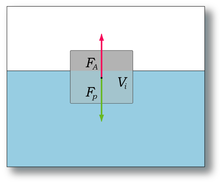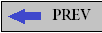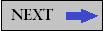# Archimedes' PrincipleArchimedes(287 BC - 212BC)

## Introduction

Archimedes' principle states that the upward buoyant force that is exerted on a body immersed in a fluid, whether fully or partially submerged, is equal to the weight of the fluid that the body displaces and acts in the upward direction at the center of mass of the displaced fluid.Archimedes' principle is a law of physics fundamental to fluid mechanics. It was formulated by Archimedes of Syracuse.

## ExplanationIn On Floating Bodies, Archimedes suggested that (c. 250 BC):

Any object, wholly or partially immersed in a fluid, is buoyed up by a force equal to the weight of the fluid displaced by the object.

Practically, Archimedes' principle allows the buoyancy of an object partially or fully immersed in a fluid to be calculated. The downward force on the object is simply its weight. The upward, or buoyant, force on the object is that stated by Archimedes' principle, above. Thus, the net force on the object is the difference between the magnitudes of the buoyant force and its weight. If this net force is positive, the object rises; if negative, the object sinks; and if zero, the object is neutrally buoyant - that is, it remains in place without either rising or sinking. In simple words, Archimedes' principle states that, when a body is partially or completely immersed in a fluid, it experiences an apparent loss in weight that is equal to the weight of the fluid displaced by the immersed part of the body.

## Formula

Consider a cuboid immersed in a fluid, with one (hence two: top and bottom) of its sides orthogonal to the direction of gravity (assumed constant across the cube's stretch). The fluid will exert a normal force on each face, but only the normal forces on top and bottom will contribute to buoyancy. The pressure difference between the bottom and the top face is directly proportional to the height (difference in depth of submersion). Multiplying the pressure difference by the area of a face gives a net force on the cuboid – the buoyancy, equaling in size the weight of the fluid displaced by the cuboid. By summing up sufficiently many arbitrarily small cuboids this reasoning may be extended to irregular shapes, and so, whatever the shape of the submerged body, the buoyant force is equal to the weight of the displaced fluid.

weight of displaced fluid = weight of object in vacuum - weight of object in fluid

The weight of the displaced fluid is directly proportional to the volume of the displaced fluid (if the surrounding fluid is of uniform density). The weight of the object in the fluid is reduced, because of the force acting on it, which is called upthrust. In simple terms, the principle states that the buoyant force (Fb) on an object is equal to the weight of the fluid displaced by the object, or the density (?) of the fluid multiplied by the submerged volume (V) times the gravity (g) or Fb = ? x g x V.Thus, among completely submerged objects with equal masses, objects with greater volume have greater buoyancy.

Suppose a rock's weight is measured as 10 newtons when suspended by a string in a vacuum with gravity acting on it. Suppose that, when the rock is lowered into water, it displaces water of weight 3 newtons. The force it then exerts on the string from which it hangs would be 10 newtons minus the 3 newtons of buoyant force: 10 - 3 = 7 newtons. Buoyancy reduces the apparent weight of objects that have sunk completely to the sea floor. It is generally easier to lift an object up through the water than it is to pull it out of the water.

For a fully submerged object, Archimedes' principle can be reformulated as follows:

apparent immersed weight = weight of object - weight of displaced fluid

then inserted into the quotient of weights, which has been expanded by the mutual volume

density of object/density of fluid = weight/weight of displaced fluid

yields the formula below. The density of the immersed object relative to the density of the fluid can easily be calculated without measuring any volume is

density of object/density of fluid = weight/weight - apparent immersed weight

(This formula is used for example in describing the measuring principle of a dasymeter and of hydrostatic weighing.)

Example: If you drop wood into water, buoyancy will keep it afloat.

Example: A helium balloon in a moving car. When increasing speed or driving in a curve, the air moves in the opposite direction to the car's acceleration. However, due to buoyancy, the balloon is pushed "out of the way" by the air, and will actually drift in the same direction as the car's acceleration.

When an object is immersed in a liquid, the liquid exerts an upward force, which is known as the buoyant force, that is proportional to the weight of the displaced liquid. The sum force acting on the object, then, is equal to the difference between the weight of the object ('down' force) and the weight of displaced liquid ('up' force). Equilibrium, or neutral buoyancy, is achieved when these two weights (and thus forces) are equal.

## Refinements

Archimedes' principle does not consider the surface tension (capillarity) acting on the body.Moreover, Archimedes' principle has been found to break down in complex fluids.

## Principle of flotation

Archimedes' principle shows the buoyant force and displacement of fluid. However, the concept of Archimedes' principle can be applied when considering why objects float. Proposition 5 of Archimedes' treatise On Floating Bodies states that:

```Any floating object displaces its own weight of fluid.
-Archimedes of Syracuse```

In other words, for an object floating on a liquid surface (like a boat) or floating submerged in a fluid (like a submarine in water or dirigible in air) the weight of the displaced liquid equals the weight of the object. Thus, only in the special case of floating does the buoyant force acting on an object equal the objects weight. Consider a 1-ton block of solid iron. As iron is nearly eight times as dense as water, it displaces only 1/8 ton of water when submerged, which is not enough to keep it afloat. Suppose the same iron block is reshaped into a bowl. It still weighs 1 ton, but when it is put in water, it displaces a greater volume of water than when it was a block. The deeper the iron bowl is immersed, the more water it displaces, and the greater the buoyant force acting on it. When the buoyant force equals 1 ton, it will sink no farther.

When any boat displaces a weight of water equal to its own weight, it floats. This is often called the "principle of flotation": A floating object displaces a weight of fluid equal to its own weight. Every ship, submarine, and dirigible must be designed to displace a weight of fluid at least equal to its own weight. A 10,000-ton ship's hull must be built wide enough, long enough and deep enough to displace 10,000 tons of water and still have some hull above the water to prevent it from sinking. It needs extra hull to fight waves that would otherwise fill it and, by increasing its mass, cause it to submerge. The same is true for vessels in air: a dirigible that weighs 100 tons needs to displace 100 tons of air. If it displaces more, it rises; if it displaces less, it falls. If the dirigible displaces exactly its weight, it hovers at a constant altitude.

While they are related to it, the principle of flotation and the concept that a submerged object displaces a volume of fluid equal to its own volume are not Archimedes' principle. Archimedes' principle, as stated above, equates the buoyant force to the weight of the fluid displaced.

One common point of confusion regarding Archimedes' principle is the meaning of displaced volume. Common demonstrations involve measuring the rise in water level when an object floats on the surface in order to calculate the displaced water. This measurement approach fails with a buoyant submerged object because the rise in the water level is directly related to the volume of the object and not the mass (except if the effective density of the object equals exactly the fluid density).

``
Thank You For Visiting My Site